## Every child is capable of learning maths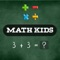# Math Kids - Math Learning game

by Hue Hoang Thi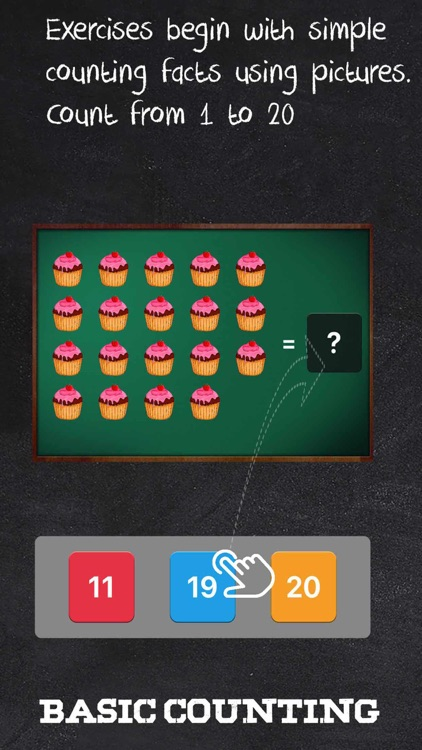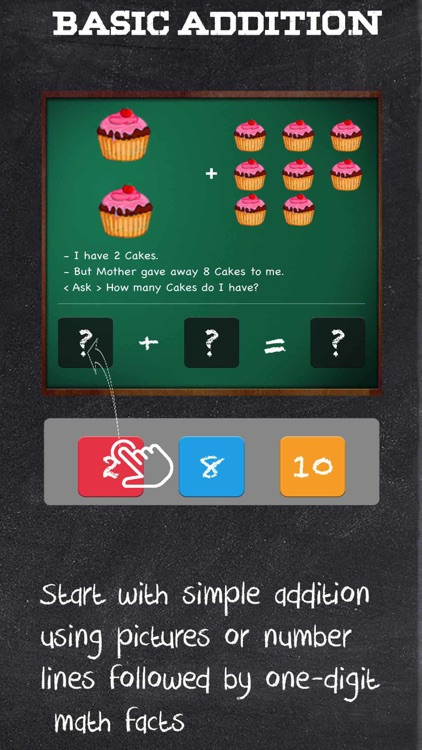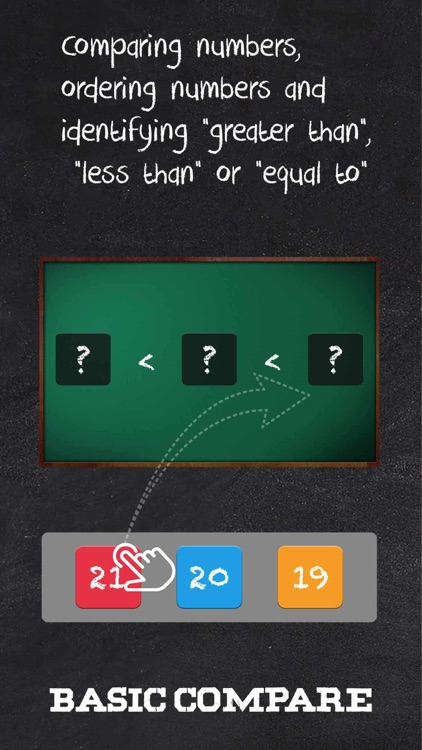Every child is capable of learning maths . So Math Kids is game activities for kids to encourage maths in early. The best way to encourage that is to share smart, well-made educational apps and games with them on a daily basis.Version
1.1
Rating
(6)
Size
66Mb
Genre
Education
Last updated
April 23, 2019
Release date
March 28, 2019

### App Screenshots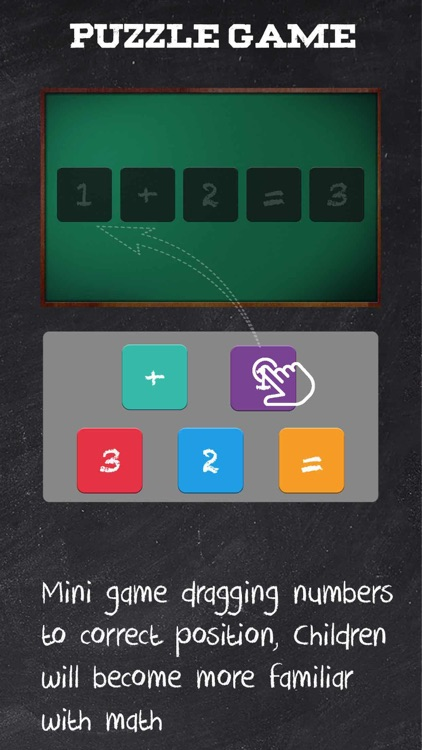### App Store Description

Every child is capable of learning maths . So Math Kids is game activities for kids to encourage maths in early. The best way to encourage that is to share smart, well-made educational apps and games with them on a daily basis.

Math Kids is game free learning to teach young children numbers and math.

Math Kids includes studies sessions, image counting, Add, Subtract, Count simple and easy to learn math.

- Counting worksheets using objects or pictures.
- Comparing numbers, ordering numbers and identifying "greater than", "less than" or "equal to". Separate worksheets with numbers up to 30 and numbers up to 100 are provided.
- Add - Start with simple addition using pictures or number lines followed by one-digit math facts and then progress through number bonds.
- Subtraction - subtraction worksheets provide practice in solving basic subtraction problems. Exercises begin with simple subtraction facts using pictures or number lines followed by 1-digit subtraction facts and and progress to subtraction of 2-digit numbers.
- Adding Puzzle - Mini game dragging numbers to correct position.
- Subtraction Puzzle - Mini game dragging numbers to correct position.

Math Kids is the perfect solution to the basics of counting, addition, and subtraction.